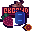# Final Countdown

Warning, the following description is pretty old. It's written so newbishly that you might lose your mind.

To avoid scrolling, the project link ishere.The project is based on the so-called UNIX time. Before I started it, I didn't even know this was a thing.

In a nutshell, UNIX measures the elapsed milliseconds since January 1st, 1970. It is the easiest to use web-time, since most languages have integrated abilities to convert the UNIX value into local and UTC time.

This project uses the current Unix time and a preset future Unix time to calculate the remaining time to said event. By using the so-called modulo, we can determine how many days, hours, minutes and seconds are left. First, we take the target time and subtract the current time. We divide the leftover by 10006060*24. Why's that? Well, one UNIX unit is one millisecond. Divide by 1000, and you get seconds. Divide by 60, and you get minutes. Again, and you get hours. Then by 24, and you got yourself the days. We then take the result and apply it to the days.

Now, the magic Modulo comes handy. For those who arent aware what that is, it basically works like division. The only difference is that it doesn't return how often the second number fits into the first, it returns the rest that cannot fit anymore. A simple example:

5 / 2 is 2.5, or 2 with a rest of 1. So, when we apply modulo: 5 % 2, we get 1 as our result. 342 % 60 = 42; 100000000037 % 100000000000 = 37 - You get the idea.

By applying Modulo with the value of the milliseconds in a day, we get the remaining hours, minutes, and seconds. We can then round this value down, and repeat the entire process with smaller values, untilwe reach the lowest possible or lowest needed value, the seconds. So, as a wrapup:

Get the remaining Unix time. Apply math to round it to the remaining number of days, then subtract the remaining number of days. Use the new value to determine remaining hours, then subtract the remaining hours. Use the new value to determine remaining minutes, subtract remainign minutes determine seconds, enter all values into the HTML, and then rinse and repeat everything all 1000ms.It appears you're using a mobile device to visit my site.

I've tried my best to make it somewhat readable, but for the best experience, visit the page on a larger screen. (min width: 750px)